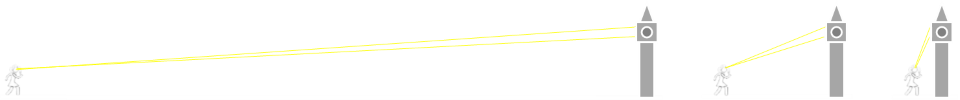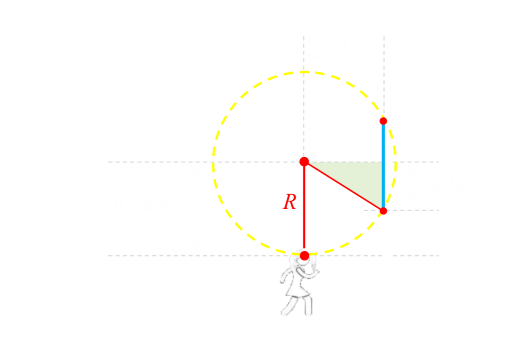# Optimal Picture Viewing Distance

A couple of weeks ago I talked about how to optimally mount a mirror so that you could see your entire body and minimize the size of the mirror needed.The solution is that you only need a mirror to be half as tall as you are to see your entire body, but it’s important where the top of the mirror is mounted. Also, we discovered, because of the way reflections work, that it does not matter how far away from the mirror you stand.
What about the optimal mounting for a picture? Something that does not reflect. What is the ‘best’ distance to stand away from a painting?
Here for ‘best’ I’m going to use the criterion of making the image subtend the broadest possible angle at the eye (appears largest).
Clearly, the further away you are from a picture, the smaller the angle it will subtend in your eye. As you get closer, the angle will get bigger. If the picture is mounted such that it spans the level of your eye, it’s obvious that the closer you get to it, the bigger it becomes (up to a theoretical limit of 180° if you slam your eyeball directly against it!)
This is the simple case.However, if the picture is mounted entirely above (or below) your level site line, then there is a distance that it is optimal to stand. Too far away and the long distance makes for very acute angle. Too close, and you’re viewing the entity at a glancing angle (also an acute viewing angle).Imagine looking at the face of a clock on the top of a tower. If you are really close, the angles are narrow.As you walk towards the tower, the subtended angle gets larger and larger, up to some maximum value, then decreases again as you get very close.

What is the optimal distance?

## Calculus

We can solve this problem using a little trigonometry, and some calculus. There’s also a neat geometric solution. We’ll look at the calculus solution first.
• We’ll define the height of the top of the picture to be a units above eye level.
• We’ll define the height of the bottom of the picture to be b units above eye level.
• The distance the viewer stands from the wall will be x units.
• The angles α and β are the angles from eye level to the respective edges of the picture.
(As before we’re assuming a flat floor, and a smooth, flat, mounting wall orthogonal to the floor).Our goal is to maximize α-β. Over the range of angles we care about, tangent is an increasing function, so all we need to do is maximize tan(α-β). This allows us to use a well known trig identity:We know the tangents of the two angles:And we can substitute these in:Differentiating this, and setting it to zero allows us to find the turning points.To make this zero, the numerator needs to be zero. Other than the trivial case when a=b, the solution is where x2=ab, or x=√(ab). The second derivative is negative, confirming that this corresponds to a maximum value turning point.
Interestingly x=√(ab) is the geometric mean of the two heights.

The optimal distance to stand away from a picture is the geometric mean of the heights of the top and bottom of the picture from your eye line.

Of course, it doesn't have to be a picture. It's the same calculation for the viewing angle of Jumbotron at a sports arena, or a movie theater (if your eye level does not split the top and bottom of the screen).## Geometric Solution

There's a solution that does not involve calculus, but it's a little more involved.
Any three points* can be used to construct a circle. We can make a circumcircle using our eyeball, and the top and bottom of the picture. The angle we're trying to determine is θ.

*As long as they are not co-linear.If you are familiar with Eulcid's Elements, specifically Proposition 20, Book III, then you will know that the inscribed angle θ from the circumference is exactly half that of the angle of the sector from the center of the circle.This means that if our goal is the maximize the angle θ, then we can maximize the center angle.
The top and the bottom of the picture are fixed, and so the way maximize the center angle is to get the center of the circle as close as possible to the wall.
The way to change the center of the circle is to change the radius of the circle. The only variable we can adjust is the position of the viewer. The distance from the viewer to the center of the circle is the radius R.The center of the circle is on a line that bisects the picture. The way to make the circle as small as possible, is for the radius to be as small as possible. The shortest distance from a point to a line is the perpendicular distance. So, geometrically, when the radius is vertical, and directly above the center of the circle, it is the shortest possible length.The circle now has the smallest possible radius, and the center is as close to the wall as possible (given the constraints that it also has to pass through the top and the bottom of the picture). The center angle is now at a maximum value, and thus, so is the inscribed angle of the viewer.There is a right triangle in the circle. Using Pythagoras:The radius is the distance from the eye to the centerline of the picture, and is the hypotenuse of this triangle. Half the height of the picture is another side of the right triangle, and the third side is the distance x that we are trying to determine. Substituting in these lengths and simplifying:And we get the same result! The distance x from the wall for the maximum angle is the geometric mean of the two heights a,b: x=√(ab).

## Try it out yourself

Here is an interactive applet that allows you to experiment with placement of the viewer, picture, and the geometry. You can drag the person left and right, and drag each edge of the picture up and down to adjust the height of the picture.
The buttons on the right toggle display of various elements of the geometry so you can follow what is going on without it being too cluttered.

## Graph

Below is a graph showing an example of how the viewing angle changes with distance from the wall.
In this example: a=10, b=4. The x-axis shows perpendicular distance from wall, x, and the y-axis shows the subtended angle (in this case in radians). Confirming our calculation, the maximum angle occurs at √40 ≈ 6.325